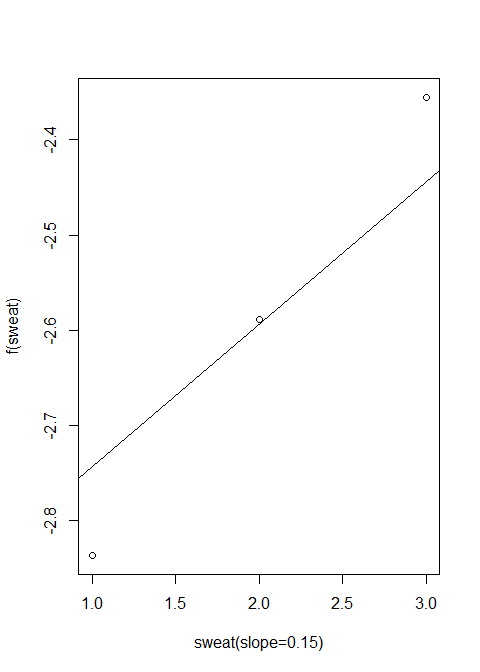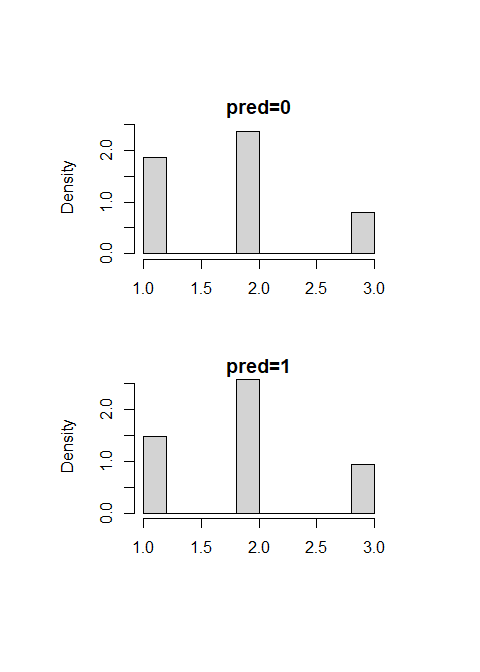Examples for Third-Variable Effect Analysis

Package installation

R package mma is used for general third-variable effect analysis . The third-variable effect (TVE) refers to the effect conveyed by intervening variables to an observed relationship between an exposure and a response variable (outcome). In this package, the exposure is also called the predictor, the intervening variables are called mediators or confounders (MC). The TVE include total effect, direct effect, and indirect effects from different third variables. The effects are defined in Yu et al. (2014). The algorithms on how to make inferences on TVEs and how to explain the effects are described in Yu et al. (2014).

To use the R package mma, we first install the package in R (install.packages("mma")) and load it.

library(mma)
#>
#> Attaching package: 'gplots'
#> The following object is masked from 'package:stats':
#>
#>     lowess
#source('C:/Users/qyu/Desktop/Research/mma/version current/R/mma.r')

Data Organization and Identify Potential Mediators/Confounders

We use weight_behavior data as an example to show how to do a preliminary data analysis to identify potential MCs and covariates. The function data.org is used for this purpose. The list of arguments for data.org are defined in . In the following example, the predictor, “pred,” is gender, and the outcome, “y,” is a binary indicator of overweight (1) or not (0). The reference group of the exposure variable is the male as defined by “predref=”M"“. There are 12 variables in”x“, which includes all potential MCs and covariates. As an argument in the function data.org,”mediator=5:12" indicates that the variables from column 5 to column 12 in x should be tested for potential MCs. Other columns in “x” are to be treated as covariates. The “mediator” argument can also be a character vector with names of the potential MCs in x. Two tests are needed to identify a potential MC: first, the variable is significantly related with the predictor adjusting for other covariates. The other covariates here is defined by “cova.” If cova is a data frame by itself, all covariates in cova are for all potential MCs. If cova is a list, the first item will be the covariate data frame and the second item list the names or column numbers of MCs in “x” that should adjust for the covariates. The significance level is set by “alpha2,” whose default value is 0.1. Second, the variable has to be significantly related with the outcome adjusting (testtype=1, by default) or not adjusting (testtype=2) for the predictor and other variables. The significance level is set by “alpha.” In the following example, p-value 1 shows the results for the second test. and p-value 2 are the results for the first test. A variable that pass the second test but not the first test is considered as covariates. Variables do not pass the second test are not adopted in further analysis. Variables in “x” but not in “mediator” are forced in further analysis as covariates.

“jointm” is a list with the first item identify the number of groups of MCs whose joint effects are of interesting, where each group is defined in an item of “jointm.” In the above example, the joint effect of Variables 5, 7, 9 is of interesting. These variables are forced into analysis as potential MCs. A variable can be shown in “jointm” multiple times. The joint effect of each group of variables will be reported. A trick to force in variables as potential MCs is to assign them in a separate group of “jointm.”

One binary/continuous predictor and one binary/continuous outcome

data("weight_behavior")
#binary predictor x
#binary y
x=weight_behavior[,c(2,4:14)]
pred=weight_behavior[,"sex"]
y=weight_behavior[,"overweigh"]
data.b.b.1<-data.org(x,y,mediator=5:12,jointm=list(n=1,j1=7:9),
pred=pred,predref="M", alpha=0.4,alpha2=0.4)
summary(data.b.b.1)
#> Identified as mediators:
#>  "tvhours"   "cmpthours" "cellhours" "exercises" "sweat"     "sports"
#> Selected as covariates:
#>  "age"       "race"      "numpeople" "car"
#> Tests:
#>             P-Value 1.y P-Value 2.pred
#> age               0.836             NA
#> race              0.850             NA
#> numpeople         0.561             NA
#> car               0.052             NA
#> gotosch           0.710             NA
#> snack             0.724             NA
#> tvhours -         0.830          0.484
#> cmpthours -       0.826          0.760
#> cellhours -       0.067          0.688
#> sports *          0.000          0.003
#> exercises *       0.176          0.203
#> sweat *           0.181          0.046
#> pred              0.178             NA
#> ----
#>  *:mediator,-:joint mediator
#>  P-Value 1:Type-3 tests in the full model (data.org) or estimated coefficients (data.org.big)
#>  when testtype=1, univariate relationship test with the outcome when testtype=2
#>  P-Value 2:Tests of relationship with the Predictor

The results from “data.org” function is summarized as above.

A potential MC defined in “mediator” argument is identified as categorical or binary if it has only two unique values or if the variable is a vector factors or characters. By default, the reference group is the first level of the variable. If the reference group needs to be changed, the categorical variable should be defined in binmed or catmed, and then the corresponding reference group in binref and catref. Continuous MCs can be defined by contmed. The following example did the same analysis as above, only that it defines that potential continuous mediators are columns 7,8,9,11, and 12 of x, binary mediators are columns 6 and 10, and categorical mediator is column 5 of x with 1 to be the reference group for all categorical and binary mediators.

data.b.b.2<-data.org(x,y,pred=pred,contmed=c(7:9,11:12),binmed=c(6,10), jointm=list(n=1,j1=7:9),
binref=c(1,1),catmed=5,catref=1,predref="M",alpha=0.4,alpha2=0.4)
summary(data.b.b.2)

Survival outcome

The functions in mma can deal with binary, categorical, continuous, or time-to-event outcomes. If the outcome is time-to-event, it should be defined by the “Surv” function in the survival package. An example of application on breast cancer survival can be found in .

cgd1<-cgd0
status<-ifelse(is.na(cgd1$etime1),0,1) y=Surv(cgd1$futime,status)
x=cgd1[,c(5:7,9:12)]
pred=cgd1[,4]
data.b.surv<-data.org(x,y,pred=pred,mediator=1:ncol(x),
alpha=0.4,alpha2=0.4)
summary(data.b.surv)

Multivariate predictors

In addition, the package can handle multivariate and multicategorical predictors. If the predictor is multicategorical of k levels, “data.org” first transform the predictor to k-1 binary predictors. If a variable significantly relates with any of the k-1 predictors, the variable passes the first test described above. P-value 2 is shown for each predictor. In the following example, the predictor is the race with six levels.

#multivariate predictor
x=weight_behavior[,c(2:3,5:14)]
pred=weight_behavior[,4]
y=weight_behavior[,15]
data.mb.b <-data.org(x,y,mediator=5:12,jointm=list(n=1,j1=c(5,7,9)),
pred=pred,predref="OTHER", alpha=0.4,alpha2=0.4)
summary(data.mb.b)
#> Identified as mediators:
#>  "tvhours"   "cellhours" "sweat"     "sports"    "gotosch"
#> Selected as covariates:
#>  "age"       "sex"       "numpeople" "car"       "exercises"
#> Tests:
#>               P-Value 1.y P-Value 2.pred P-Value 2.predAFRICAN
#> age                 0.836             NA                    NA
#> sex                 0.178             NA                    NA
#> numpeople           0.561             NA                    NA
#> car                 0.052             NA                    NA
#> gotosch -           0.710          0.112                 0.796
#> snack               0.724             NA                    NA
#> tvhours -           0.830          0.748                 0.535
#> cmpthours           0.826             NA                    NA
#> cellhours -         0.067          0.880                 0.994
#> sports *            0.000          0.587                 0.163
#> exercises           0.176          0.629                 0.731
#> sweat *             0.181          0.647                 0.174
#> pred                0.543             NA                    NA
#> predAFRICAN         0.663             NA                    NA
#> predCAUCASIAN       0.242             NA                    NA
#> predINDIAN          0.890             NA                    NA
#> predMIXED           0.782             NA                    NA
#>               P-Value 2.predCAUCASIAN P-Value 2.predINDIAN P-Value 2.predMIXED
#> age                                NA                   NA                  NA
#> sex                                NA                   NA                  NA
#> numpeople                          NA                   NA                  NA
#> car                                NA                   NA                  NA
#> gotosch -                       0.996                0.097               0.033
#> snack                              NA                   NA                  NA
#> tvhours -                       0.334                0.916               0.092
#> cmpthours                          NA                   NA                  NA
#> cellhours -                     0.707                0.555               0.383
#> sports *                        0.816                0.453               0.091
#> exercises                       0.723                0.912               0.881
#> sweat *                         0.214                0.374               0.203
#> pred                               NA                   NA                  NA
#> predAFRICAN                        NA                   NA                  NA
#> predCAUCASIAN                      NA                   NA                  NA
#> predINDIAN                         NA                   NA                  NA
#> predMIXED                          NA                   NA                  NA
#> ----
#>  *:mediator,-:joint mediator
#>  P-Value 1:Type-3 tests in the full model (data.org) or estimated coefficients (data.org.big)
#>  when testtype=1, univariate relationship test with the outcome when testtype=2
#>  P-Value 2:Tests of relationship with the Predictor

Multivariate outcomes

Similarly, the package can deal with multivariate outcomes. The following code deals with multivariate predictors and multivariate responses. If the variable is significantly related with any one of the outcomes, it passes the second test described above. The results from “data.org” are summarized for each combination of the exposure-outcome relationship.

#multivariate responses
x=weight_behavior[,c(2:3,5:14)]
pred=weight_behavior[,4]
y=weight_behavior[,c(1,15)]
data.mb.mb<-data.org(x,y,mediator=5:12,jointm=list(n=1,j1=c(5,7,9)),
pred=pred,predref="OTHER", alpha=0.4,alpha2=0.4)
summary(data.mb.mb)
#> Identified as mediators:
#>  "tvhours"   "cellhours" "sweat"     "sports"    "gotosch"
#> Selected as covariates:
#>  "age"       "sex"       "numpeople" "car"       "exercises"
#> Tests:
#>               P-Value 1.bmi P-Value 1.overweigh P-Value 2.pred
#> age                   0.458               0.836             NA
#> sex                   0.003               0.178             NA
#> numpeople             0.282               0.561             NA
#> car                   0.059               0.052             NA
#> gotosch -             0.527               0.710          0.112
#> snack                 0.830               0.724             NA
#> tvhours -             0.505               0.830          0.748
#> cmpthours             0.676               0.826             NA
#> cellhours -           0.084               0.067          0.880
#> sports *              0.001               0.000          0.587
#> exercises             0.089               0.176          0.629
#> sweat *               0.078               0.181          0.647
#> pred                  0.344               0.543             NA
#> predAFRICAN           0.900               0.663             NA
#> predCAUCASIAN         0.032               0.242             NA
#> predINDIAN            0.446               0.890             NA
#> predMIXED             0.833               0.782             NA
#>               P-Value 2.predAFRICAN P-Value 2.predCAUCASIAN
#> age                              NA                      NA
#> sex                              NA                      NA
#> numpeople                        NA                      NA
#> car                              NA                      NA
#> gotosch -                     0.796                   0.996
#> snack                            NA                      NA
#> tvhours -                     0.535                   0.334
#> cmpthours                        NA                      NA
#> cellhours -                   0.994                   0.707
#> sports *                      0.163                   0.816
#> exercises                     0.731                   0.723
#> sweat *                       0.174                   0.214
#> pred                             NA                      NA
#> predAFRICAN                      NA                      NA
#> predCAUCASIAN                    NA                      NA
#> predINDIAN                       NA                      NA
#> predMIXED                        NA                      NA
#>               P-Value 2.predINDIAN P-Value 2.predMIXED
#> age                             NA                  NA
#> sex                             NA                  NA
#> numpeople                       NA                  NA
#> car                             NA                  NA
#> gotosch -                    0.097               0.033
#> snack                           NA                  NA
#> tvhours -                    0.916               0.092
#> cmpthours                       NA                  NA
#> cellhours -                  0.555               0.383
#> sports *                     0.453               0.091
#> exercises                    0.912               0.881
#> sweat *                      0.374               0.203
#> pred                            NA                  NA
#> predAFRICAN                     NA                  NA
#> predCAUCASIAN                   NA                  NA
#> predINDIAN                      NA                  NA
#> predMIXED                       NA                  NA
#> ----
#>  *:mediator,-:joint mediator
#>  P-Value 1:Type-3 tests in the full model (data.org) or estimated coefficients (data.org.big)
#>  when testtype=1, univariate relationship test with the outcome when testtype=2
#>  P-Value 2:Tests of relationship with the Predictor

##Third-Variable Effect Analysis Next, we use med function to estimate the TVE using the results from data.org function. The default approach is using a generalized linear model to fit the final full model. If “nonlinear=TRUE”", multiple Additive Regression Trees (MART) will be used to fit the relationship between outcome(s) and other variables, and smoothing splines used to fit the relationship between the potential MC and exposure variables(s).

Binary predictor and binary outcome

med.b.b.2<-med(data=data.b.b.2,n=2,nonlinear=TRUE)

Using the linear method and to show the results:

med.b.b.1<-med(data=data.b.b.2,n=2)
med.b.b.1
#>
#>
#> For the predictor pred :
#>  The estimated total effect: 0.6075
#>
#>  The estimated indirect effect:
#>       y1.all   y1.tvhours y1.cmpthours y1.cellhours y1.exercises     y1.sweat
#>       0.2028       0.0117       0.0222       0.0358      -0.0272       0.0110
#>    y1.sports        y1.j1
#>       0.1276       0.0064

We can plot the marginal effect of the MC on the outcome, and the marginal effect of the predictor on the MC. If the predictor is binary, plot.med draws a histogram or boxplot of the marginal density of the MC at each different value of the predictor.

plot(med.b.b.1, data.b.b.2,vari="exercises",xlim=c(0,50))
plot(med.b.b.2, data.b.b.2,vari="sports")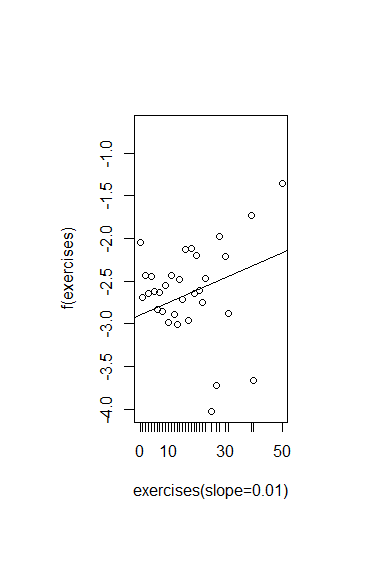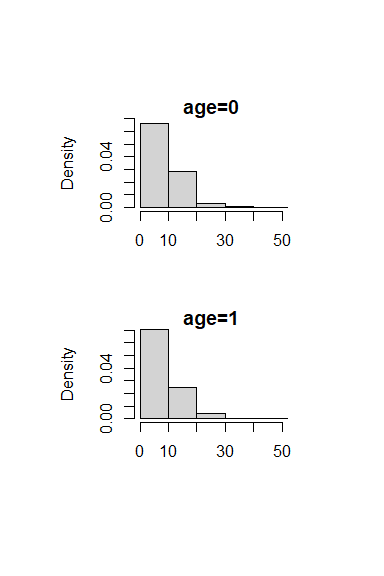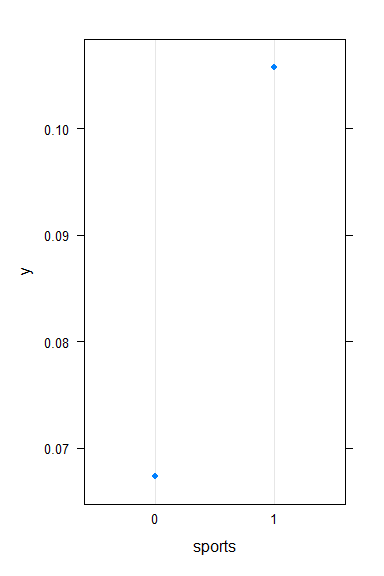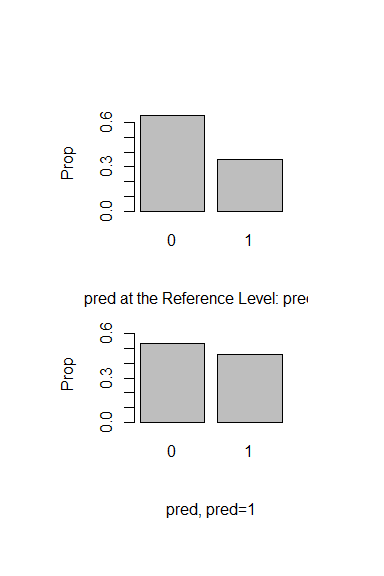Survival outcome

For survival outcome, the default option is to fit the final full model using Cox proportional hazard model. Note that when use type=“lp,” the linear predictor is the type of predicted value as in predict.coxph, the results is similar to those from MART. Readers are referred to for more details.

med.b.surv.1<-med(data=data.b.surv,n=2,type="lp")
#close to mart results when use type="lp"
med.b.surv.2<-med(data=data.b.surv,n=2,nonlinear=TRUE)
#results in the linear part unit

Statistical Inference on TVE Analysis

boot.med function is used to make inferences on the TVE. Results return from data.org function is input as the first argument.

Binary predictor and binary outcome

bootmed.b.b.1<-boot.med(data=data.b.b.2,n=2,n2=50)
#>  1
#>  2
#>  3
#>  4
#>  5
#>  6
#>  7
#>  8
#>  9
#>  10
#>  11
#>  12
#>  13
#>  14
#>  15
#>  16
#>  17
#>  18
#>  19
#>  20
#>  21
#>  22
#>  23
#>  24
#>  25
#>  26
#>  27
#>  28
#>  29
#>  30
#>  31
#>  32
#>  33
#>  34
#>  35
#>  36
#>  37
#>  38
#>  39
#>  40
#>  41
#>  42
#>  43
#>  44
#>  45
#>  46
#>  47
#>  48
#>  49
#>  50
summary(bootmed.b.b.1)
#> MMA Analysis: Estimated Mediation Effects Using GLM
#> For Predictor/Moderator at pred
#> $total.effect #> est mean sd upbd lwbd upbd_q lwbd_q upbd_b lwbd_b p_norm p_quan #> 0.470 0.724 0.389 1.486 -0.038 1.454 -0.020 1.493 -0.176 0.063 0.080 #> #>$direct.effect
#>    est   mean     sd   upbd   lwbd upbd_q lwbd_q upbd_b lwbd_b p_norm p_quan
#>  0.384  0.478  0.359  1.183 -0.226  1.154 -0.104  1.282 -0.246  0.183  0.240
#>
#> $indirect.effect #> y1.all y1.tvhours y1.cmpthours y1.cellhours y1.exercises y1.sweat #> est 0.087 0.017 0.028 -0.008 -0.014 -0.003 #> mean 0.246 -0.012 0.028 0.036 -0.011 0.013 #> sd 0.143 0.037 0.052 0.064 0.023 0.039 #> upbd 0.526 0.062 0.131 0.162 0.033 0.090 #> lwbd -0.035 -0.085 -0.075 -0.089 -0.056 -0.064 #> upbd_q 0.572 0.061 0.177 0.175 0.020 0.094 #> lwbd_q 0.058 -0.072 -0.069 -0.082 -0.072 -0.054 #> upbd_b 0.690 0.070 0.190 0.181 0.035 0.096 #> lwbd_b -0.009 -0.111 -0.085 -0.114 -0.083 -0.057 #> p_norm 0.086 0.758 0.594 0.571 0.617 0.743 #> p_quan 0.040 0.640 0.440 0.560 0.680 0.840 #> y1.sports y1.j1 #> est 0.094 0.028 #> mean 0.172 0.062 #> sd 0.089 0.099 #> upbd 0.346 0.255 #> lwbd -0.003 -0.131 #> upbd_q 0.378 0.267 #> lwbd_q 0.039 -0.083 #> upbd_b 0.425 0.271 #> lwbd_b -0.017 -0.095 #> p_norm 0.054 0.530 #> p_quan 0.040 0.560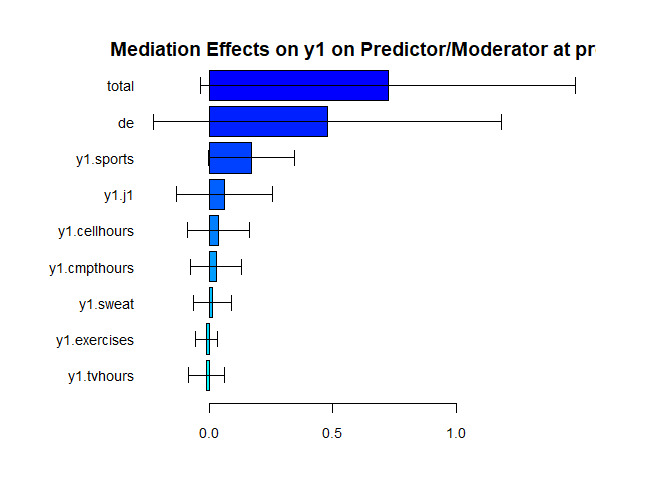Similarly, the default approach is using a generalized linear model to fit the final full model. If the argument nonlinear=TRUE, multiple Additive Regression Trees (MART) will be used to fit the model in estimating the outcome. Applications for nonlinear Mediation Analysis are described in Yu et al. (2017, 2018). bootmed.b.b.2<-boot.med(data=data.b.b.2,n=2,n2=40,nu=0.05,nonlinear=TRUE) summary(bootmed.b.b.2) #> MMA Analysis: Estimated Mediation Effects Using MART #> For Predictor/Moderator at pred #>$total.effect
#>    est   mean     sd   upbd   lwbd upbd_q lwbd_q upbd_b lwbd_b p_norm p_quan
#>  0.085  0.296  0.238  0.762 -0.170  0.610  0.115  0.278  0.112  0.213  0.000
#>
#> $direct.effect #> est mean sd upbd lwbd upbd_q lwbd_q upbd_b lwbd_b p_norm p_quan #> 0.012 0.205 0.281 0.755 -0.346 0.570 -0.007 0.211 -0.009 0.466 0.500 #> #>$indirect.effect
#>        y1.all y1.tvhours y1.cmpthours y1.cellhours y1.exercises y1.sweat
#> est     0.073      0.012        0.004        0.000       -0.014    0.009
#> mean    0.091      0.006        0.009        0.001        0.014   -0.002
#> sd      0.048      0.010        0.006        0.006        0.016    0.017
#> upbd    0.185      0.026        0.022        0.013        0.045    0.032
#> lwbd   -0.002     -0.013       -0.003       -0.010       -0.017   -0.036
#> upbd_q  0.139      0.013        0.018        0.008        0.029    0.020
#> lwbd_q  0.039     -0.007        0.005       -0.006       -0.001   -0.019
#> upbd_b  0.140      0.013        0.008        0.003        0.029    0.022
#> lwbd_b  0.067     -0.008        0.005       -0.007       -0.001   -0.020
#> p_norm  0.055      0.534        0.128        0.816        0.366    0.909
#> p_quan  0.000      0.500        0.000        0.500        0.500    0.500
#>        y1.sports  y1.j1
#> est        0.072  0.018
#> mean       0.073  0.008
#> sd         0.053  0.013
#> upbd       0.178  0.032
#> lwbd      -0.032 -0.017
#> upbd_q     0.142  0.024
#> lwbd_q     0.033 -0.002
#> upbd_b     0.147  0.026
#> lwbd_b     0.035  0.002
#> p_norm     0.172  0.551
#> p_quan     0.000  0.500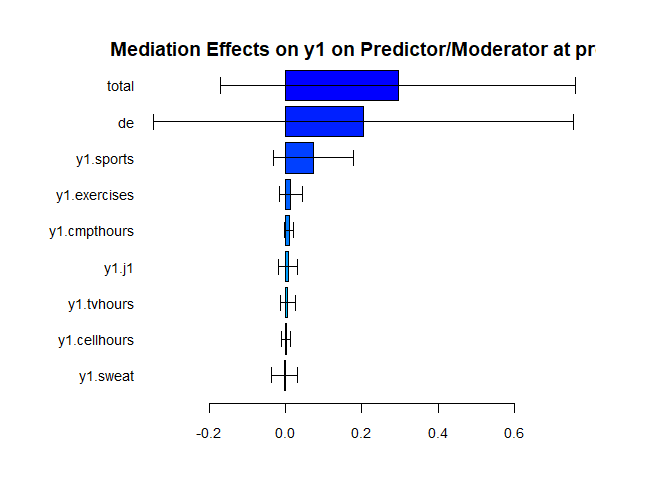Plots of the fitted mma object from boot.med

plot.mma() plots the marginal effect of the selected variable on the outcome, and the marginal effect of the predictor on the selected variable. Interpretations for similar plots can be found in .

plot(bootmed.b.b.1,vari="exercises",xlim=c(0,50))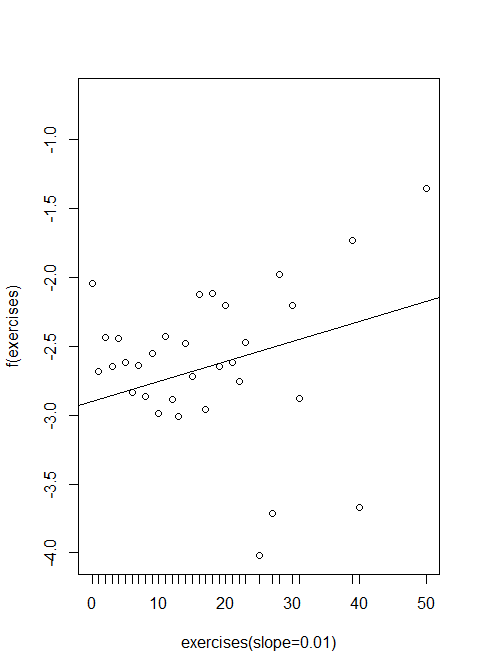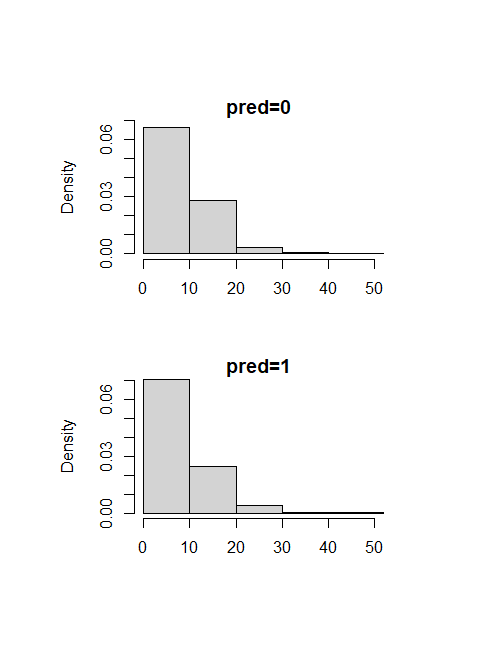plot(bootmed.b.b.1,vari="sports")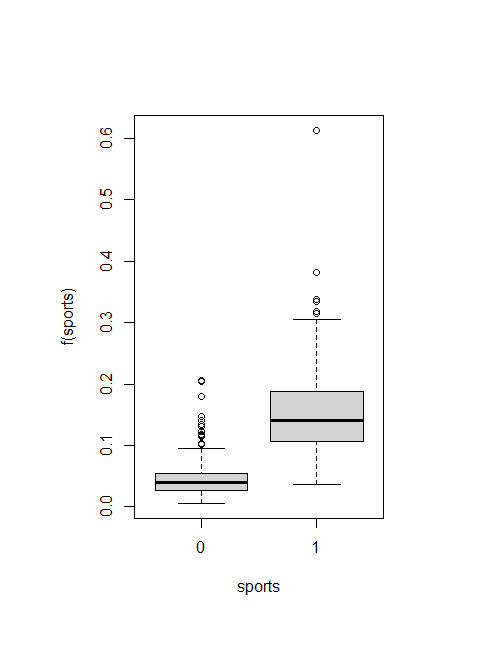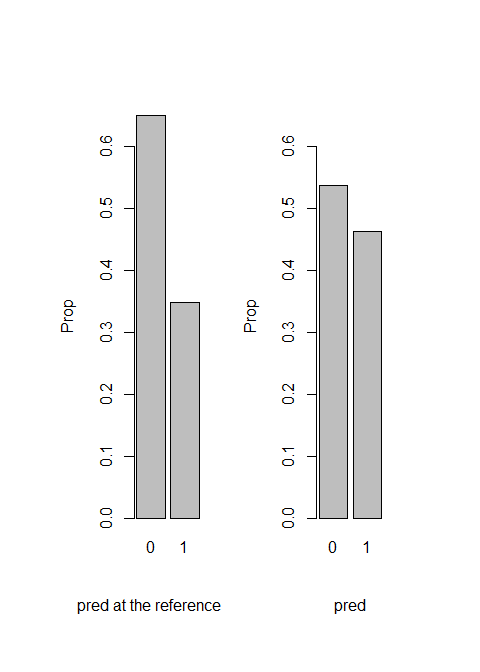Combined function for multiple TVE analysis

The mma function is a combined function that automatically identify potential MCs, based on which to make statistical inference on the mediation effects.

x=weight_behavior[,c(2,4:14)]
pred=weight_behavior[,3]
y=weight_behavior[,15]
mma.b.b.glm<-mma(x,y,pred=pred,contmed=c(7:9,11:12),binmed=c(6,10),binref=c(1,1), catmed=5,catref=1,predref="M",alpha=0.4,alpha2=0.4,n=2,n2=2)
#>  1
#>  2
summary(mma.b.b.glm)
#> MMA Analysis: Estimated Mediation Effects Using GLM
#> For Predictor/Moderator at pred
#> $total.effect #> est mean sd upbd lwbd upbd_q lwbd_q upbd_b lwbd_b p_norm p_quan #> 0.573 0.452 0.332 1.103 -0.200 0.675 0.228 0.686 0.686 0.174 0.000 #> #>$direct.effect
#>    est   mean     sd   upbd   lwbd upbd_q lwbd_q upbd_b lwbd_b p_norm p_quan
#>  0.410  0.213  0.488  1.170 -0.744  0.541 -0.115  0.558  0.558  0.663  1.000
#>
#> $indirect.effect #> y1.all y1.exercises y1.sweat y1.sports #> est 0.163 -0.019 0.021 0.155 #> mean 0.239 0.008 0.015 0.234 #> sd 0.156 0.026 0.007 0.183 #> upbd 0.545 0.059 0.029 0.593 #> lwbd -0.067 -0.044 0.001 -0.125 #> upbd_q 0.344 0.026 0.020 0.357 #> lwbd_q 0.134 -0.010 0.010 0.111 #> upbd_b 0.129 0.129 0.129 0.129 #> lwbd_b -0.011 -0.011 -0.011 -0.011 #> p_norm 0.126 0.765 0.040 0.201 #> p_quan 0.000 1.000 0.000 0.000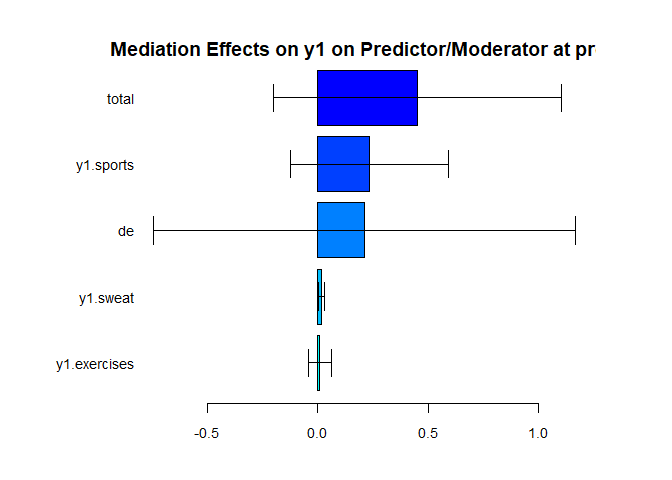When the argument nonlinear=TRUE, multiple Additive Regression Trees (MART) will be used to fit the final full model in estimating the outcome. mma.b.b.mart<-mma(x,y,pred=pred,contmed=c(7:9,11:12),binmed=c(6,10),binref=c(1,1), catmed=5,catref=1,predref="M",alpha=0.4,alpha2=0.4,nonlinear=TRUE,n=2,n2=5) summary(mma.b.b.mart) summary(mma.b.b.mart) #> MMA Analysis: Estimated Mediation Effects Using MART #> For Predictor/Moderator at pred #>$total.effect
#>    est   mean     sd   upbd   lwbd upbd_q lwbd_q upbd_b lwbd_b p_norm p_quan
#>  0.056  0.260  0.219  0.689 -0.170  0.533  0.033  0.399  0.029  0.236  0.000
#>
#> $direct.effect #> est mean sd upbd lwbd upbd_q lwbd_q upbd_b lwbd_b p_norm p_quan #> 0.026 0.120 0.149 0.411 -0.171 0.271 -0.039 0.272 -0.041 0.420 0.800 #> #>$indirect.effect
#>        y1.all y1.exercises y1.sweat y1.sports
#> est     0.030       -0.004    0.002     0.044
#> mean    0.140        0.005    0.013     0.114
#> sd      0.085        0.025    0.015     0.077
#> upbd    0.307        0.054    0.041     0.265
#> lwbd   -0.027       -0.044   -0.016    -0.037
#> upbd_q  0.269        0.021    0.027     0.230
#> lwbd_q  0.072       -0.034   -0.009     0.051
#> upbd_b  0.132        0.022    0.017     0.114
#> lwbd_b  0.070        0.010   -0.011     0.050
#> p_norm  0.101        0.833    0.389     0.139
#> p_quan  0.000        0.400    0.400     0.000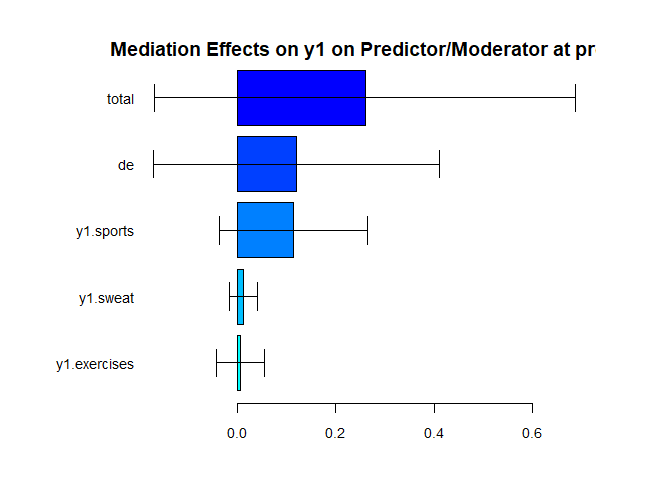plot(mma.b.b.mart,vari="exercises")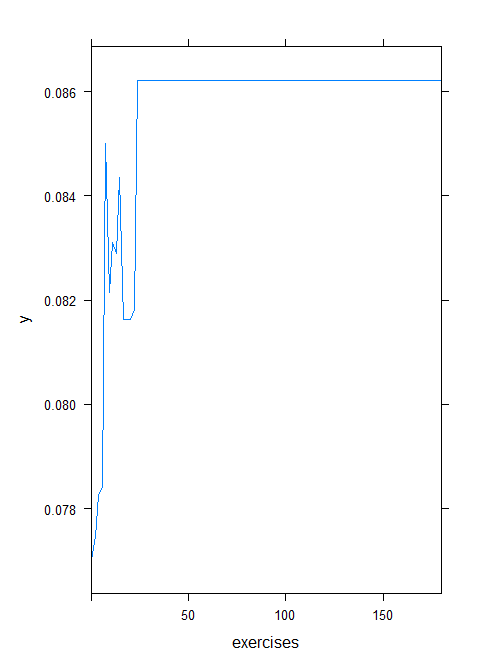#> Error in pred_name[, z.b]: incorrect number of dimensionsplot(mma.b.b.glm,vari="sweat")